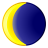# Plotting points along a line

615
2
05-08-2014 06:52 AMNew Contributor
Trying to plot station points along a line at intermittent distances.

For example:

If the line is 200m, I want ten station points.
The first and last station point need to be 10m from the start and end.
Points in between need to be spaced 20m apart.

start |----------O--------------------O-------------------O   --->> continuing along 200m

Have tried the 'Create Points Along Line' tool, but unable to manipulate for varying distances.

Would like a 'batchable' model.

Does anybody have a suggestion with how to proceed?

Thanks
Tags (3)
2 RepliesbyMVP Honored Contributor
Trying to plot station points along a line at intermittent distances.

For example:

If the line is 200m, I want ten station points.
The first and last station point need to be 10m from the start and end.
Points in between need to be spaced 20m apart.

start |----------O--------------------O-------------------O   --->> continuing along 200m

Have tried the 'Create Points Along Line' tool, but unable to manipulate for varying distances.

Would like a 'batchable' model.

Does anybody have a suggestion with how to proceed?

Thanks

Convert the lines to routes with the Create Routes tool and using M values based on length.  I would then create an Excel spreadsheet that copied every RouteID and route length from the routes using copy/paste from the Route Table View.  The station formula just involves duplicating the RouteID and length inserted 9 times below the original route entry and entering 10 and 30 in the first two rows and then pulling the pattern down to the last row so that it ends on the value 190.  I don't know what parts of this pattern you intend to retain for any other lines of other lengths.  Is 20m more important than 10 divisions or the other way around?  In any case it is easy enough to manipulate a variety of patterns for each RouteID in Excel if you have the line length.  If 10 divisions is more important it is relatively easy to make an Excel formula to use the length value and Row number to do the division amounts.  (Convert formulas to values before using the spreadsheet in ArcMap).  Then import the Excel spreadsheet into ArcMap and make it an event table to create a Route Event Layer of points.Occasional Contributor III
An alternative might be to create points along the line using this geoprocessing tool:
ftp://lnnr.lummi-nsn.gov/GIS_Scripts/CreatePointsAlongALine/

Make the points with a 10 meter spacing.  The output features will be points on your line attributed with distance from the start node.   The tool even would with lines with bends.

Next, this tool will populate the resulting point file with a new attribute with a modulo value.
ftp://lnnr.lummi-nsn.gov/GIS_Scripts/Modulo/

Given your example you calculate a modulo value of 20 on the distance values.  Using the new modulo values, select any point with a modulo other than zero and you will get a selection of the points at 10m, 30m, 50m from the start node.

Of course, this works for one line of length 200m, or for all the lines in your line feature class with length of 200m.  If you have many lines of different lengths, and you have Python or Modelbuilder skills, you could iterate the lines, get the lengths, calculate the division disance, and run the process using the values from each iteration.

Good luck!